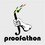# USAMO 1997 Problem 5

Problem 5 from the 1997 USAMO seemed easy. Do you think my proof is correct?

Let $s=a^3+b^3+c^3+abc$ and $f(x)=\frac{1}{s-x^3}$. Since the terms in the inequality are homogeneous, assume without loss of generality that $a+b+c=\sqrt{s}$, such that $0\lt a,b,c\lt\sqrt{s}$. For all $0\lt x\lt\sqrt{s}$, $f(x)$ is convex by the second derivative test. We have $f(a)+f(b)+f(c)\le3f(\frac{a+b+c}{3})=\frac{81}{26s}$ by Jensen's Inequality. To prove that $\frac{81}{26(a^3+b^3+c^3+abc)}\le\frac{1}{abc}$, we have $\frac{81}{26}\le\frac{a^3+b^3+c^3+abc}{abc}$ and $\frac{81}{26}-4\le0\le\frac{a^3+b^3+c^3-3abc}{abc}=\frac{(a+b+c)((a-b)^2+(a-c)^2+(b-c)^2)}{2abc}$Note by Cody Johnson
6 years, 9 months ago

This discussion board is a place to discuss our Daily Challenges and the math and science related to those challenges. Explanations are more than just a solution — they should explain the steps and thinking strategies that you used to obtain the solution. Comments should further the discussion of math and science.

When posting on Brilliant:

• Use the emojis to react to an explanation, whether you're congratulating a job well done , or just really confused .
• Ask specific questions about the challenge or the steps in somebody's explanation. Well-posed questions can add a lot to the discussion, but posting "I don't understand!" doesn't help anyone.
• Try to contribute something new to the discussion, whether it is an extension, generalization or other idea related to the challenge.

MarkdownAppears as
*italics* or _italics_ italics
**bold** or __bold__ bold
- bulleted- list
• bulleted
• list
1. numbered2. list
1. numbered
2. list
Note: you must add a full line of space before and after lists for them to show up correctly
paragraph 1paragraph 2

paragraph 1

paragraph 2

[example link](https://brilliant.org)example link
> This is a quote
This is a quote
    # I indented these lines
# 4 spaces, and now they show
# up as a code block.

print "hello world"
# I indented these lines
# 4 spaces, and now they show
# up as a code block.

print "hello world"
MathAppears as
Remember to wrap math in $$ ... $$ or $ ... $ to ensure proper formatting.
2 \times 3 $2 \times 3$
2^{34} $2^{34}$
a_{i-1} $a_{i-1}$
\frac{2}{3} $\frac{2}{3}$
\sqrt{2} $\sqrt{2}$
\sum_{i=1}^3 $\sum_{i=1}^3$
\sin \theta $\sin \theta$
\boxed{123} $\boxed{123}$

Sort by:

God I wish I knew how to do problems like this...

- 6 years, 9 months ago

Answer You are absolutely right ...

- 6 years, 9 months ago

Yeah,no flaws.Great work.

- 6 years, 9 months ago

Can you explain the WLOG? In particular, if $a=b=c$, what would the WLOG values be?

Staff - 6 years, 9 months ago

Do you need to explain your choice of WLOG on these exams?

Well, if it holds for $\{a,b,c\}$ such that $a+b+c=\sqrt{s}$, we can show it holds for $\{at,bt,ct\}$ where $t$ is a positive real:

$\sum_{cyc}\frac{1}{(at)^3+(bt)^3+abct^3}\le\frac{1}{abct^3}$

Multiplying by $t^3$ gives the desired result. So, for a set $\{a',b',c'\}$, let $p=a'+b'+c'$. If we multiply both sides by $\frac{\sqrt{s}}{p}$, we have $\{at,bt,ct\}=\{a',b',c'\}$ with $t=\frac{p}{\sqrt{s}}$, so it holds for all positive real $\{a,b,c\}$.

- 6 years, 9 months ago

Yes, you have to explain the WLOG, and make sure that it indeed is WLOG. Your current application is not standard or typical, and you should review how to apply it properly. The reason I'm pointing it out, is that WLOG does not hold, but you have instead restricted the possible sets.

Note that you defined the variable $s$ twice, and need both scaled equations to hold. You have not answered how to deal with the case that $a = b = c =1$. What is the scaling value of $t$ and the corresponding value of $s$?

Staff - 6 years, 9 months ago

OH I SEE WHAT I DID WRONG. Because it should be a constant, not a variable, for WLOG.

- 6 years, 8 months ago

It's a valid proof, but I would argue that the second derivative test isn't necessarily fair game on the USAMO.

- 6 years, 9 months ago

I am pointing out that this is not a valid proof. Review the WLOG argument, in which he actually introduces a restrictive condition.

The second derivative test (for convexity) is fair game on the USAMO. However, make sure that you write out the full statements, instead of just claiming that it works (as was done above).

Staff - 6 years, 9 months ago